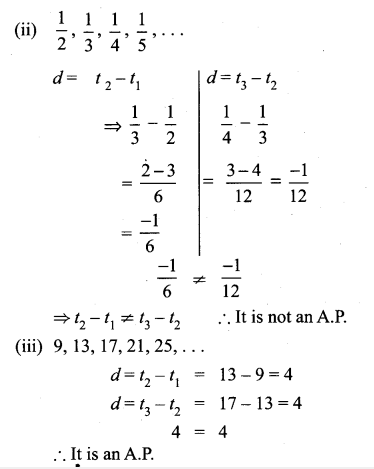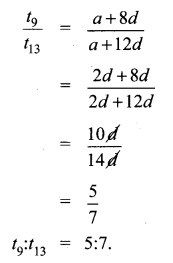# Tamilnadu Board Class 10 Maths Solutions Chapter 2 Numbers and Sequences Ex 2.5

## Tamilnadu State Board Class 10 Maths Solutions Chapter 2 Numbers and Sequences Ex 2.5

Question 1.
Check whether the following sequences are in A.P.
(i) a – 3, a – 5, a – 7,…
(ii) $$\frac { 1 }{ 2 }$$,$$\frac { 1 }{ 3 }$$,$$\frac { 1 }{ 4 }$$,$$\frac { 1 }{ 4 }$$,………..
(iii) 9, 13, 17, 21, 25,…
(iv) $$\frac { -1 }{ 3 }$$,0,$$\frac { 1 }{ 3 }$$,$$\frac { 2 }{ 3 }$$,………..
(v) 1,-1, 1,-1, 1,-1,…
Solution:
To prove it is an A.P, we have to show d = t2 – t1 = t3 – t2.
(i) a – 3, a – 5, a – 7………
t1,t2,t3(v) 1,-1, 1,-1, 1,-1,…
d = t2 – t1 = -1 -1 = -2
d = t3 – t2 = 1 – (-1) = 2
-2 ≠ 2 ∴ It is not an A.P.

Question 2.
First term a and common difference d are given below. Find the corresponding A.P.
(i) a = 5,d = 6
(ii) a = 7,d = 5
(iii) a = $$\frac { 3 }{ 4 }$$, d = $$\frac { 1 }{ 2 }$$
Solution:
(i) a = 5,d = 6
A.P a, a + d, a + 2d,….
= 5,5 + 6,5 + 2 × 6,…
= 5,11,17,…
(ii) a = 7,d = -5
A.P. = a,a + d,a + 2d,…
= 7,7 + (-5), 7 + 2(-5),…
= 7,2,-3,…
(iii) a = $$\frac { 3 }{ 4 }$$, d = $$\frac { 1 }{ 2 }$$Question 3.
Find the first term and common difference of the Arithmetic Progressions whose nth terms are given below
(i) tn = -3 + 2n
(ii) tn = 4 – 7n
Solution:
(i) a = t1 = -3 + 2(1) = -3 + 2 = -1
d = t2 – t1
Here t2 = -3 + 2(2) = -3 + 4 = 1
∴ d = t2 – t1 = 1 – (-1) = 2
(ii) a = t1 = 4 – 7(1) = 4 – 7 = -3
d = t2 – t1
Here t2 = 4 – 7(2) = 4 – 14 – 10
∴ d = t2 – t1 = 10 – (-3) = -7

Question 4.
Find the 19th term of an A.P. -11, -15, -19,…
A.P = -11,-15,-19,…
a = -11
d = t2 – t1 =-15-(-11)
= -15 + 11
= -4
n = 19
∴ tn = a + (n – 1)d
t19 = -11 + (19 – 1)(-4)
= -11 + 18 × -4
= -11 – 72
= -83

Question 5.
Which term of an A.P. 16,11, 6,1,… is -54 ?
A.P = 16, 11,6, 1,…
It is given that
tn = -54
a = 16, d = t2 – t1 = 11 – 16 = -5
∴ tn = a + (n – 1)d
-54 = 16 + (n – 1) (-5)
-54 = 16 – 5n + 5
21 – 5n = -54
-5n = -54 -21
-5n = -75
n = $$\frac { 75 }{ 5 }$$ =15
∴ 15th term is -54.

Question 6.
Find the middle term(s) of an A.P. 9, 15, 21, 27,…,183.
Solution:
A.P = 9, 15,21,27,…, 183
No. of terms in an A.P. is
n = $$\frac { l-a }{ d }$$ + 1
a = 9, l = 183, d = 15 – 9 = 6
∴ n = $$\frac { 183-9 }{ 6 }$$ + 1
= $$\frac { 174 }{ 6 }$$ + 1
= 29 + 1 = 30
∴ No. of terms = 30. The middle must be 15th term and 16th term.
∴ t15 = a + (n – 1)d
= 9 + 14 × 6
=9 + 84
= 93
t16 = a + 15 d
= 9 + 15 × 6
= 9 + 90 = 99
∴ The middle terms are 93, 99.

Question 7.
If nine times ninth term is equal to the fifteen times fifteenth term, show that six times twenty fourth term is zero.
Solution:
Nine times ninth term = Fifteen times fifteenth term
9t9 = 15t15
9(a + 8d) = 5(a + 14d)
9a + 72d = 15a + 210
15a + 210d – 9a – 72d = 0
⇒ 6a + 138 d = 0
⇒ 6(a + 23 d) = 0
⇒ 6(a + (24 – 1)d) = 0
⇒ 6t24 = 0. Hence it is proved.

Question 8.
If 3 + k, 18 – k, 5k + 1 are in A.P. then find k.
Solution:
3 + k, 18 – k, 5k + 1 are in A.P
⇒ 2b = a + c if a, b, c are in A.PQuestion 9.
Find x, y and z, given that the numbers x, 10, y, 24, z are in A.P.
Solution:
A.P = x, 10, y, 24, z,…
d = t2 – t1 = 10 – x …(1)
= t3 – t2 = y – 10 …(2)
= t4 – t3 = 24 – y …(3)
= t5 – t4 = z – 24 ….(4)
(2) and (3)
⇒ y – 10 = 24 – y
2y = 24 + 10 = 34
y = $$\frac { 34 }{ 2 }$$ = 17From (3) and (4)
24 – y = z – 24
24 – 17 = z – 24
7 = z – 24
∴ z = 7 + 24 = 31
∴ Solutions x = 3
y = 17
z = 31

Question 10.
In a theatre, there are 20 seats in the front row and 30 rows were allotted. Each successive row contains two additional seats than its front row. How many seats are there in the last row?
Solution:
t1 = a = 20
t2 = a + 2 = 22
t3 = a + 2 + 2 = 24 ⇒ d = 2
∴ There are 30 rows.
t30 = a + 29d
= 20 + 29 × 2
= 20 + 58
= 78
∴ There will be 78 seats in the last row.

Question 11.
The sum of three consecutive terms that are in A.P. is 27 and their product is 288. Find the three terms.
Solution:
Let the three consecutive terms be a – d, a, a + dQuestion 12.
The ratio of 6th and 8th term of an A.P is 7:9. Find the ratio of 9th term to 13th term.
Solution:
$$\frac{t_{6}}{t_{8}}=\frac{7}{9}$$
$$\frac { a+5d }{ a+7d }$$ = $$\frac { 7 }{ 9 }$$
9a + 45d = 7a + 49d
9 a + 45 – 7d = 7a + 49 d
9a + 45d – 7a – 49d = 0
2a – 4d = 0 ⇒ 2a = 4d
a = 2d
Substitue a = 2d inQuestion 13.
In a winter season let us take the temperature of Ooty from Monday to Friday to be in A.P. The sum of temperatures from Monday to Wednesday is 0° C and the sum of the temperatures from Wednesday to Friday is 18° C. Find the temperature on each of the five days.
Solution:
Let the five days temperature be (a – d), a, a + d, a + 2d, a + 3d.
The three days sum = a – a + a + a + a = 0
⇒ 3a = 0 ⇒ a = 0. (given)
a + d + a + 2d + a + 3d = 18
3a + 6d = 18
3(0) + 6 d = 18
6d = 18
d = $$\frac { 18 }{ 6 }$$ = 3
∴ The temperature of each five days is a – d, a, a + d, a + 2d, a + 3d
0 – 3, 0, 0 + 3, 0 + 2(3), 0 + 3(3)
= -3°C, 0°C, 3°C, 6°C, 9°C

Question 14.
Priya earned ₹ 15,000 in the first month. Thereafter her salary increased by ₹1500 per year. Her expenses are ₹13,000 during the first year and the expenses increases by ₹900 per year. How long will it take for her to save ₹20,000 per month.
Solution:We find that the yearly savings is in A.P with a1 = 2000 and d = 600.
We are required to find how many years are required to save 20,000 a year …………..
an = 20,000
an = a + (n – 1)d
20000 = 2000 + (n – 1)600
(n – 1)600 = 18000
n – 1 = $$\frac { 18000 }{ 600 }$$ = 30
n = 31 years